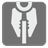Robot Sort
Elementary+
English RU

The spaceship reactors contains several (no more than ten) nuclear fuel rods. These rods should be sorted by size to ensure the reactor’s stability, but as usual the fuel rod loading mechanism malfunctioned and inserted the rods at random, then started the ships engine. Now Stephan has to reload the rods all over again. Because the reactor is already running, he needs to quickly swap neighbouring rods or else the whole thing goes BOOM!

You are given the sizes and initial order of the rods as an array of numbers. Indexes are position, values are sizes. You should order this array from the smallest to the largest in size.

For each action Stephan can swap only two neighbouring elements. Each action should be represented as a string with two digits - indexes of the swapped elements (ex, "01" - swap 0th and 1st rods). The result should be represented as a string that contains the sequence of actions separated by commas. If the array does not require sorting, then return an empty string.

And you can swap only N*(N-1)/2 times, where N - is a quantity of rods.Input: An array as a tuple of integers.

Output: The sequence of actions as a string.

Example:

```swapsort((6, 4, 2)) == "01,12,01"
swapsort((1, 2, 3, 4, 5)) == ""
swapsort((1, 2, 3, 5, 3)) == "43"
```

How it is used: This mission will show you how to work the simplest sorting algorithms. And this can be useful, if you need to build a production line for your factory.

Precondition:
1 ≤ len(array) ≤ 10
all(1 ≤ n < 10 for n in array)